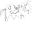# Seating Charts

Creating a seating chart for a class is an extremely interesting problem that delves into the realms of both web design and competitive programming.

#### ### Overview

There are two questions I attempted to answer.

1. How to create an easy to use interface that minimizes the cognitive load on the user
2. How to best create the seating chart given a list of preferences for each student

#### ### Algorithmn

The second question was significantly more interesting.

In order to simplify the process, I further broke this problem down into two subproblems. Intuitively, students interact the most with the people at their table the most. Thus, I first attempted to choose the table groups, and then assigned seats.

To quantify the “goodness” of a particular table group, I calculated the fraction of the people that each person listed down as “preferred” who were at the table. More formally,

``````studentScore = preferences
.map(name => group.includes(name) ? 1: 0)
.reduce((a, b) => a + b, 0);
``````

From personal experience, it can be really hard to work in a table group where you don’t know anybody. Thus, I added a significant penalty if none of the person’s listed preferences existed at the table.

``````if(studentScore === 0) studentScore = -100;
``````

Note that because the maximum score for any student is 1, this is an extremely harsh penalty. Thus, our algorithmn will always seek to minimize the number of such “lonely” people.

Summing across all `studentScore`s resulted in the overall score for the table group.

This problem can be approached with a dynamic programming or DP solution. A DP solution breaks down the problem into smaller subproblems. It then caches the solutions, resulting in significantly reduced overhead. In general, the crucial element of dynamic programming is the “state” that we DP on, or the representation of the problem. I chose to represent my state as an N element array, where the ith position represents if we’ve used the ith element.

Note that the score for a state can be calculated by first selecting a subset representing a table group, and then calculating the subproblem after removing the subset we chose. This results in an `O(2^N * N^K)` where N is the number of students and K is the number of students per table group. Admittedly, this is not a very good runtime but in practice we can optimize away much of the computation.

#### ### Considerations

Some interesting things I noted.

1. Many of the table groups tended towards entirely male or female.
2. Multiple different seating charts would not vary the table groups greatly.

These appear to be problems inherent in allowing students to list their own preferences. The better we are at listening to the students, the more they can introduce their own biases (such as with gendered tables). In addition, there are very few optimal solutions so our algorithmn would naturally tend toward the same few seating charts in an attempt to best listen to the listed preferences.